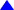Econ 2630                           Study Guides: Ch 16 thru 18                         Dr. Usip

Study Guide for Ch. 16

1.    List the three important macroeconomic goals, and explain why each is important.

2.    Explain how economists track economic growth using real GDP.

3.    Discuss the four phases of the business cycle.

4.    Explain why macroeconomists use aggregation.

5.    Explain why macroeconomists sometimes disagree with each other.

Study Guide for Ch 17

1.    State the definition of Gross Domestic Product (GDP) and describe the problems with and limitations of this measure.

2.    Explain why it is important to take inventory changes into account when measuring GDP.

3.    Explain why actual private investment spending might not equal planned private investment spending.

4.    Define net investment spending and explain why it is not a perfect measure of the increase in the capital stock in a given year.

5.    List the three categories of non-business production that add to the nation’s capital stock, but that are not counted as private investment.

6.    Explain why transfer payments are not included in our measure of GDP.

7.    Explain the difference between the expenditure, value-added, and factor payments approaches to GDP, and describe how GDP is calculated using each approach.

8.    Explain the difference between real and nominal GDP.

9.    Discuss the usefulness of GDP figures in the short run and the long run.

10.    Describe the types of unemployment and relate them to the concept of full employment.

11.    Discuss the costs of unemployment.

12.    Explain how the unemployment rate is measured, and address the limitations of this measure.

Study Guide for Ch. 18

1.    Name and describe the two functions of a monetary system.

2.    Explain why paper currency is accepted as a means of payment.

3.    Describe how index numbers are calculated.

4.    Discuss how the Consumer Price Index (CPI) is compiled and how it is used.

5.    Describe what the inflation rate measures.

6.    Define deflation.

7.    Explain why it is important to translate nominal variables into real variables.

8.    Discuss why the GDP price index, rather than the CPI, is used to translate nominal GDP into real GDP.

9.    Describe the costs associated with inflation, and explain why inflation—by itself—does not erode the average real income in the economy.

10.    Explain why inflation can redistribute purchasing power when it is not correctly
anticipated.

11.    Explain why the CPI overstates the inflation rate, and why this is a problem.

12.    Describe the steps the Bureau of Labor Statistics (BLS) has taken to make the CPI more accurate.

13.    Describe the problems inherent in using the CPI to measure the cost of living.Top or Return to Course Information or Home Page or Send me Comments via E-mail